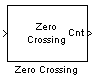# Zero Crossing

Count number of times signal crosses zero in single time step

•Libraries:
DSP System Toolbox / Signal Operations

## Description

The Zero Crossing block concludes that a signal in a given channel has passed through zero if it meets any of the following criteria, where xi is the current value of the signal, xi−1 is the previous value, and so on:

• xi < 0 and xi−1 > 0

• xi > 0 and xi−1 < 0

• For a positive integer L, xi < 0, xi−1 = 0, and xiL−1 > 0, where $0\le l\le L$.

• For a positive integer L, xi > 0, xi−1 = 0, and xiL−1 < 0, where $0\le l\le L$.

For the first value of the input signal, xi−1 and xi−2 are zero. The block outputs the number of times the signal crosses zero in a single time step at the Cnt port.

## Ports

### Input

expand all

The input signal specified as a real-valued vector or matrix.

Data Types: `single` | `double` | `int8` | `int16` | `int32` | `uint8` | `uint16` | `uint32` | `fixed point`

### Output

expand all

The block outputs the number of zero crossings in the data input as one of these:

• Scalar –– When the data input is a column vector, the block outputs a scalar value representing the number of zero crossings in the data input.

• Row vector –– When the data input is a matrix, the block outputs a row vector with each element in the row vector representing the number of zero crossings in the corresponding column of the data input.

Data Types: `uint32`

## Parameters

expand all

Specify how the block should process the input.

• `Columns as channels (frame based)` — When you select this option, the block treats each column of the input as a separate channel.

• `Elements as channels (sample based)` — When you select this option, the block treats each element of the input as a separate channel.

## Block Characteristics

 Data Types `double` | `fixed point` | `integer` | `single` Direct Feedthrough `no` Multidimensional Signals `no` Variable-Size Signals `no` Zero-Crossing Detection `no`

## Version History

Introduced before R2006a